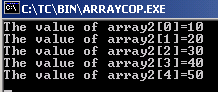Tutorials

# C Array copy example

The example below contains two arrays: array1 and array2 with sizes 5 respectively.

The example below contains two arrays: array1 and array2 with sizes 5 respectively.

# C Array copy example

In this section, you will learn how to copy an array in C.

The example below contains two arrays: array1 and array2 with sizes 5 respectively. Here the size of an array states its capacity to hold maximum number of values.

Now in the example each element of array1 is assigned a value. Then, in order to copy the elements of array1 into array2, we have used for loop with which the values located at the indexes of array1 will be as it is get copied and pasted at the same indexes of array2.

Here is the code:

ARRAYCOP.C

 ``` #include  #include  int main() {   clrscr();   int array1;   int array2;   int i, j;   array1=10;   array1=20;   array1=30;   array1=40;   array1=50;   for (i=0; i<5; i++)   array2[i]=array1[i];   for (j=0; j<5; j++)   printf("The value of %d=%d\n", j, array2[j]);   getch();   return 0; }```

The array is copied consisting of same elements:

ARRAYCOP.EXE